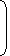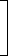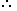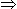# Tricks to solve Aptitude Problems – Profit and LossIMPORTANT FACTS

Cost Price:

The price, at which an article is purchased, is called its cost price, abbreviated as C.P.

Selling Price:

The price, at which an article is sold, is called its selling prices, abbreviated as S.P.

Profit or Gain:

If S.P. is greater than C.P., the seller is said to have a profit or gain.

Loss:

If S.P. is less than C.P., the seller is said to have incurred a loss.

IMPORTANT FORMULAE

1. Gain = (S.P.) – (C.P.)
2. Loss = (C.P.) – (S.P.)
3. Loss or gain is always reckoned on C.P.
4. Gain Percentage: (Gain %)
 Gain % =Gain x 100C.P.
5. Loss Percentage: (Loss %)
 Loss % =Loss x 100C.P.
6. Selling Price: (S.P.)
 SP =(100 + Gain %) x C.P100
7. Selling Price: (S.P.)
 SP =(100 – Loss %) x C.P.100
8. Cost Price: (C.P.)
 C.P. =100 x S.P.(100 + Gain %)
9. Cost Price: (C.P.)
 C.P. =100 x S.P.(100 – Loss %)
10. If an article is sold at a gain of say 35%, then S.P. = 135% of C.P.
11. If an article is sold at a loss of say, 35% then S.P. = 65% of C.P.
12. When a person sells two similar items, one at a gain of say x%, and the other at a loss of x%, then the seller always incurs a loss given by:
 Loss % =Common Loss and Gain %2 =x2 . 10 10
13. If a trader professes to sell his goods at cost price, but uses false weights, then
 Gain % =Error x 100%. (True Value) – (Error)

GENERAL QUESTIONS

Alfred buys an old scooter for Rs. 4700 and spends Rs. 800 on its repairs. If he sells the scooter for Rs. 5800, his gain percent is:
A.
 4 4 % 7
B.
 5 5 % 11
C. 10%
D. 12%

Answer: Option B

Explanation:

Cost Price (C.P.) = Rs. (4700 + 800) = Rs. 5500.

Selling Price (S.P.) = Rs. 5800.

Gain = (S.P.) – (C.P.) = Rs.(5800 – 5500) = Rs. 300.

 Gain % =300 x 100% = 5 5 % 5500 11
The cost price of 20 articles is the same as the selling price of x articles. If the profit is 25%, then the value of x is:
 A. 15 B. 16 C. 18 D. 25

Answer: Option B

Explanation:

Let C.P. of each article be Re. 1 C.P. of x articles = Rs. x.

S.P. of x articles = Rs. 20.

Profit = Rs. (20 – x).20 – x x 100 = 25x2000 – 100x = 25x

125x = 2000x = 16.

#### If selling price is doubled, the profit triples. Find the profit percent.

Correct! Wrong!

#### In a certain store, the profit is 320% of the cost. If the cost increases by 25% but the selling price remains constant, approximately what percentage of the selling price is the profit?

Correct! Wrong!

#### A vendor bought toffees at 6 for a rupee. How many for a rupee must he sell to gain 20%?

Correct! Wrong!

#### The percentage profit earned by selling an article for Rs. 1920 is equal to the percentage loss incurred by selling the same article for Rs. 1280. At what price should the article be sold to make 25% profit?

Correct! Wrong!

#### A shopkeeper expects a gain of 22.5% on his cost price. If in a week, his sale was of Rs. 392, what was his profit?

Correct! Wrong!

QUIZ ON PROFIT & LOSS
GREAT JOB!!!

Share your Results:

Don't miss out!
Subscribe To Our Newsletter

Learn new things. Get an article everyday.

Invalid email address
Give it a try. You can unsubscribe at any time.